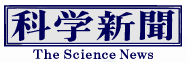Latest News# Successful multiplication using an optical quantum computer: Realization of non-linear measurement of an optical field by RIKEN and the University of Tokyo

2023.08.25

An international joint research group led by Postdoctoral Researcher Atsushi Sakaguchi at RIKEN Center for Quantum Computing (RQC) and Team Leader Akira Furusawa (Professor of the School of Engineering at the University of Tokyo) has realized the first-ever non-linear measurement of an optical field. This measurement corresponds to non-linear calculations that enable universal quantum computation in optical quantum computers. Sakaguchi said, "The fact that we were able to demonstrate non-linearity with light paves the way for a universal quantum computer. This system can also be used for error correction." Furusawa added, "We hope to develop a practical optical quantum computer within the current fiscal year." The research results were published in Nature Communications.

The optical quantum computer is one of the many candidates for quantum computers and offers advantages such as room-temperature operation, high-speed capabilities, and high compatibility with optical communications. However, since quantum manipulation is performed by continuously measuring light in a quantum entangled state, the operations on the optical field are limited to addition, subtraction, and constant multiplication. To perform arbitrary calculations, it is essential to perform multiplication operations between optical fields, which requires non-linear measurements.

A theoretical proposal to realize non-linear measurement was made in 2001, but the proposal employed hypothetical particles. The research group proposed a specific setup in 2016. Non-linear measurements are realized as follows: after interference of the measured light with auxiliary quantum light, one of the two resulting lights is measured; then, phase rotation operations are dynamically applied, based on the non-linear calculation results, to the other light before measurement.

However, synchronizing the non-linear calculation with the optical circuit has been a challenge. For example, since light travels approximately 300 m in 1000 ns, delaying it by 300 meters using optical cables would introduce significant errors and make synchronization difficult. In this study, the research group addressed this issue by incorporating non-linear calculation into memory blocks within programmable digital circuits using lookup tables (calculation tables). This allowed the calculation results to be read out in a time period as short as 2.67 ns. By changing the calculation table written into the lookup table, it is also possible to vary the type of calculation while maintaining the calculation time.

Input/output signals for measurement and control systems are analog signals; therefore, conversion is necessary to interface these systems with digital circuits. By developing a dedicated conversion board, the total delay time, including the conversion process, was reduced to 26.8 ns.

For the constructed experimental setup, weak laser light with 27 intensity levels and random phases was input in 2.16 million combinations and measured to verify the relationship between the input light and measurement results. The results showed that the component of input light with the same phase as the reference phase of the measuring instrument varied quadratically, while the orthogonal phase component yielded proportional measurement values. Additionally, the fidelity of the Wigner function for the changed quantum state was confirmed to be 0.99 or higher, and the reduction in measurement noise achieved using non-linear squeezed light as an auxiliary photon was found to align with theoretical values. These results demonstrated that the constructed experimental setup successfully achieved the goal of non-linear measurements.

The success of this non-linear measurement opens up possibilities for universal calculations, including multiplication, in optical measurement-based quantum computing. Furthermore, the combination of auxiliary quantum light and non-linear feedforward can be directly applied to error-resistant calculations.

Furusawa said, "Computer science relies on the existence of practical computers. Therefore, we aim to develop a practical optical quantum computer and make it accessible to numerous researchers and students on the cloud, fostering quantum computer science. Quantum computers can solve only limited problems rapidly, but for any computation, increasing the clock speed will enhance the processing speed. Leveraging the properties of optical quantum computers, our future goal is to achieve a 100 GHz, 100-core super quantum computer."

Journal Information
Publication: Nature Communications
Title: Nonlinear feedforward enabling quantum computation
DOI: 10.1038/s41467-023-39195-w

This article has been translated by JST with permission from The Science News Ltd. (https://sci-news.co.jp/). Unauthorized reproduction of the article and photographs is prohibited.

Latest News